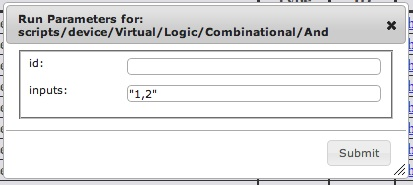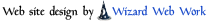Scripts << ScriptsRunning Software Overview  |  Sitemap  |  Downloads  |  Developers  |  ForumsHome Devices FAQs How-Tos Projects Tutorials Recipes

# Adding Devices - Running ScriptsScripts are commonly used to add a device into the system. Another common use is to set up the system (devices, device configuration, wiring).

To run a Script, find its name on the Scripts page and click on the "Run" action to the right of it. After clicking, you will usually be presented with a parameters dialog box asking you to fill in any parameters the Script may need. Some Scripts, (e.g., some Scripts in the "examples" area), need no parameters and will run without showing the parameters box.

Most Scripts will need parameters. Device Scripts and their parameters are more fully described in the Devices section. Information about a Script and its parameters is also available inside a Script. Instead of clicking on a Script's "Run" action, click on the "Show" action and read through the Script. Lines starting with a '#' are comment lines and describe what the Script does and it parameters.

## Script ParametersMost Scripts take a set of parameters, and when a user runs a Script, the system will query the user for the parameters using a dialog box. In the illustration above, the user has run the Script for an And device, and the system is asking the user to define the "id" and "inputs" parameters.

After the user submits the parameters, behind the scenes, the system will submit these parameters to a Script interpreter. All Script parameters must be interpretable by the Script interpreter.

The Script interpreter understands the following value types: numbers (e.g. 1,2,1.0,-2,..), `true`, `false`, `nil`, commands, and double quoted strings. Any Script parameter must be one of these. Generally speaking, Script parameters will not be commands, so Script parameters will be one of the remaining options.

`true`, `false`, and `nil` mean just what they say and are useful when a parameter should be one of these qualities.

If a parameter is not `true`, `false`, or `nil`, likely it is a number or a double quoted string. A double quoted string is any text string with double quotes surrounding it. So "hi", "1,2,3", "my_device" are all valid double quoted strings.

So if a Script parameter is looking for a pin name:

pin_name: ....

It's looking for a name, which, given the possible choices, has to be a double quoted string. So the parameter could be `"pin_name"`, but not `pin_name` since `pin_name` will not be understood by the interpreter (note: unquoted strings are interpreted as commands, and the interpreter won't know what a `pin_name` command is).

If a Script parameter is looking for pin numbers:

pin_numbers: ...

It's looking for a number (1,2,3, ...) or a set of numbers. Typing a single number (e.g. `123`) will work. If one wants to supply a set of numbers, the obvious thing to type `1,2,3` will not work. It's not a number or a double quoted string. Typing `"1,2,3"` will work.

When supplying a parameter, make sure you supply a number, `true`, `false`, `nil`, or a double quoted string.

## Some Interesting Script Parameters

### Device IDs

As mentioned earlier in this section, device Scripts are described in the Devices section and within the Script itself. However, one parameter is common to all device Scripts and it is best described here. The parameter is the "id" parameter. IDs are user defined names assigned to devices. They can be any name as long as the name starts with a letter and is followed by letters, numbers or underscores. Valid device IDs are "Switch", "happy_day", "light2". Invalid IDs are "1switch", "_switch", or "happy day". Since an ID is a device name, no devices can have the same name. IDs are case sensitive.

In some of the web screens, screens for viewing or wiring devices as examples, devices will be identified by their IDs. Also, when running a Script which alters a device in some way (say adding a pin to a device), the Script will need a device ID parameter. The device ID parameter identifies which device is being modified, and the parameter is the ID parameter of the device. So device IDs both identify a device, and they are parameters for other Scripts specifying which device the Scripts will act upon.

### Function and Scaling Parameters (less common)

Sometimes it is necessary to take a number on a device terminal and change it into another number. For example, perhaps a terminal has a light intensity in Lux (with a max of 500), and we want to convert it into in a percentage value between 0 and 100. One could create a new device (using Ruby code) for doing this, but this is a common enough occurrence that there is an easier way to do it.

Some devices take a "function" or "scaling" parameter which defines a formula for changing their starting numeric output value into another. So for example, a function parameter which inverts an output value would look like:

`function: "1/x"`

As seen above, inversion is cased by dividing 1 by the initial output (1/x). The "x" value is a place holder representing the initial value supplied to the function. It could be any unknown string ("y", "z", "a", "b"). Functions work by performing the known mathematical operations (+,-,\,* etc.) on the numbers, constants, and unrecognized strings in the function, substituting the initial value in for any unrecognized strings. So to get back to our original problem of converting Lux (max of 500) to percentage (0..100):

`function: "round(lux / 500 * 100)"`

This function introduces an internal function "round" which takes a floating point number and rounds it to the nearest integer. Functions work in floating point, so results which are not whole numbers will be returned as floating point numbers, unless they are rounded. The "lux" string is an unknown string, so it becomes the input value for the function.

Taking the problem a step further, if we wanted to get a percentage value with one digit of resolution after the decimal point:

`function: "round(lux / 500 * 100 * 10) / 10"`

Function/scaling parameters support the following operators, functions, and predefined constants.

Operators:

• `+ : adds 2 numbers`
• `- : subtracts 2 numbers, or if in front of a number, negates the number`
• `* : multiplies 2 numbers`
• `/ : divides 2 numbers`
• `** : exponential function. 2**3 raises 2 to the power 3 (8)`

Functions:

• `ln() : takes the natural log of a number`
• `log() : takes the log base 10 of a number`
• `rand() : generates random numbers within a range*`
• `round() : rounds a number to the nearest integer`
• `() : evaluates whatever is inside and returns a number`

Constants:

• `E : the mathematical constant "e"`
• `PI : the mathematical constant "pi"`

Examples of other formulas:

• `"2 * PI * r"` - circumference of a circle in terms of its radius (r)
• `"PI * r**2"` - area of a circle in terms of its radius (r)
• `"round((F - 32) * 5 / 9)"` - converting Fahrenheit to Celsius

The rand() function takes a single "max value" integer parameter which must be greater than or equal to 0. If the value is 0, it will generate random floating point values greater than or equal to 0.0 and less than 1.0. For all other integer values, it will generate random integers starting at 0 and less than the "max value" integer. A side effect of this definition is that rand(1) is not very interesting - it will always return 0.

The rand() function is useful if you want to create a Device which generates pseudo data for testing. Using the Mapper Device and the rand() function, you can create Devices which generate data within any range you choose.

For example, here is a Device which generates floating point output data from 90.0 to 94.9:

`````` run_script("Scripts/Device/Virtual/Mapper", function:"90 + rand(50)/10.0", id:"rand_900_949")
``````

## Catalina Computing, LLC.